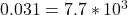## Two particles, with identical positive charges and a separation of 2.65 10-2 m, are released from rest. Immediately after the release, parti

Question

Two particles, with identical positive charges and a separation of 2.65 10-2 m, are released from rest. Immediately after the release, particle 1 has an acceleration 1 whose magnitude is 5.00 103 m/s2, while particle 2 has an acceleration 2 whose magnitude is 7.70 103 m/s2. Particle 1 has a mass of 6.20 10-6 kg. (a) Find the charge on each particle. q1 = C q2 = C (b) Find the mass of particle 2. kg

in progress 0
2 months 2021-07-22T14:15:42+00:00 1 Answers 2 views 0

a)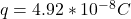b)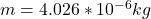Explanation:

From the question we are told that:

Separation distance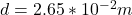Acceleration 1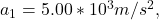Acceleration 2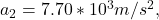Mass 1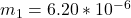Generally the equation for Force is mathematically given by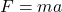For Particle 1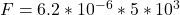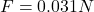Also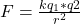Where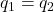Therefore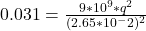b)

Generally the equation for Force is mathematically given by